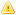Home |Sign in | EnglishHelpWLanguageWLanguage functionsStandard functionsNumeric values management functionsMathematical functionsArcTanPresentationExampleHow do you find an angle using its tangent?See alsoAbsArcCosArcSinArcTan2ArcTanCoTanConversionCosDecimalPartDecimalToSexagesimalExpFactorialIntegerPartIsEvenIsOddLnLogMathematical functionsPowerRootRoundRoundDownRoundUpSexagesimalToDecimalSinTangentThis content has been translated automatically. Click here to view the French version.
 How do you find an angle using its tangent? WINDEVWEBDEVWINDEV MobileOthersArcTan (Function) In french: ArcTang Returns the arc tangent of a numeric value (integer or real).Example // Récupération de l'arc tangente de 12ResTang = ArcTan(12)// Renvoie 85,2363583093 Syntax = ArcTang() : RealArc tangent (angle in degrees of the tangent) of the specified numeric value, included in the [-90, 90] interval (excluding bounds). : Integer or realNumeric value (tangent) to use. Remarks How do you find an angle using its tangent?In a right triangle, the tangent of an angle is equal to the ratio of the opposite side to the adjacent side. How do I find the angle? Simply use ArcTan. Example: The following code: Trace("ArcTang(1) = " + ArcTan(1))Trace("ArcTang(3/4) = " + ArcTan(3/4)) displayed in the trace window: ArcTang(1) = 45ArcTang(3/4) = 36.86989764584 Component: wd270mat.dll Minimum version required Version 9 This page is also available for…